# How to use RANKX in DAX (Part 2 of 3 – Calculated Measures)

The first article in this series looked at to use the RANKX function in a calculated column to apply ranking to your data. This article will show how you can use the RANKX function in a calculated measure.

I will use the same 10-row data-set and provide various types of ranking over the My Value column.

 Category Sub Category Date My Value A A1 2018-01-01 2 A A2 2018-01-02 4 A A3 2018-01-03 6 A A4 2018-01-04 6 B B1 2018-01-05 21 B B2 2018-01-06 22 B B2 2018-01-07 23 C C1 2018-01-08 35 C C2 2018-01-09 35 C C3 2018-01-10 35

To quickly add this table to your data model, create a new calculated table in your data model using the following code.

## How RANKX works

The syntax for RANKX is as follows:

#### RANKX(<table>, <expression>[, <value>[, <order>[, <ties>]]])

The details of the syntax were explained in the first article, and is exactly the same regardless of using in a calculated column or measure.  A quick summary is the function will loop as many times as there are rows in the <table> passed as the first parameter.  The RANKX function will return a  single value that represents the order in which the <expression> occurs in the the list of values generated during the loop.

### Ranking a Category

First, lets demonstrate an example for readers just wanting to see something that works.  The following code will show the rank of the Category data based on the SUM of the My Value column.  The calculated measure is:

When this  calculated measure is added to a visual along with the ‘Table'[Category] and ‘Table'[My Value] fields. You should see the following.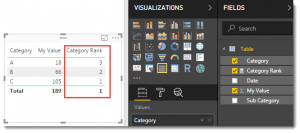The numbers in the Category Rank column, correctly rank Categories A, B & C based on the SUM of My Value.  Additional parameters of RANKX can be used to switch the ranking order.  This parameter was explained in part 1.

### Deconstruction of calculation

There are a couple of important aspects to this code.  The first is the use of the ALL function in line 3, while the second is the use of the CALCULATE function.

Lets look at what the ALL function is doing first.

The ALL function in DAX is useful for two things.  It can be used to clear filters from a filter context as part of a complex calculation, but it can also be used to generate and return a table.  This is how the ALL function is being used.  The ALL function is being used to return a table to be used as the first parameter of the RANKX function.

All the AggX (Iterator or X) functions require a table to be used as the first parameter.  Every AggX function will loop a series of iterations based on the number of rows in the table passed as the first argument.  In this example, the first argument uses the ALL function over a column.

Used this way, the ALL function returns a single column table with all the distinct values from the column.  If you would like to see the actual data the ALL function returns, just create a calculated table using just that code (as follows).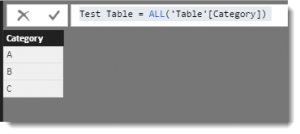The output of this is a single column, three row table.  This means the RANKX function for our example will iterate three times.  Once for every row in the table being generated by the ALL function.

The second parameter in the RANKX function is the <expression>.  The expression is logically executed as many times as there are loops in the iterator (or rows in the table used in the first parameter).  The output of the <expression> is not the final output of the RANKX function, rather it is used as an intermediary step.

It is helpful to understand what each iteration of <expression> generates, to understand what the RANKX function is doing to determine its final output.  This is where another AggX function can help.

### Using CONCATENATEX to help debug

I’m going to reach out to a friend in another AggX function to help visualise what is happening.  Lets introduce another calculated measure using the CONCATENATEX function to help.  The new calculated measure is as follows.

The only difference between this and the RANKX calculated measure from earlier is it’s using the CONCATENATEX function instead of RANKX.  There is also a third parameter to introduce commas to separate the values.

CONCATENATEX  simply builds up a string which is added to each time the AggX function performs an iteration.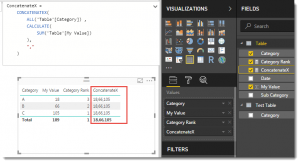The output of the [ConcatenateX] calculated measure gives us an insight into how many loops were iterated as well as showing us the output for each iterator <expression>.

The top row of the fourth column shows text (18,66,105)  that is the result of the AggX function running three loops.  The first loop executed the <expression> and produced a value of 18.  The second iteration executed the <expression> and produced a value of 66, finally the third loop executed the <expression> and produced a value of 105.

The reason why the <expression> produced a different value (18,66 & 105)  is because the CALCULATE function used in each iteration <expression> converted row context from the visual to filter context.  I will look at this in more depth later in this article.  This column shows the combined output of the <expression> generated by each loop of the AggX function.

Where the RANKX function differs from CONCATENATEX, is rather than simply adding values to a string, the function sorts the values (18 for “A”, 66 for “B” and 105 for “C”), and then compares the result of a separate execution of <expression> for the position the current row of the visual would appear in that sorted list.

The result of <expression> for the top row (Category A) of the visual happens to be 18, which is item 3 in the sorted list.  The result of <expression> for the second row (Category B) is 66, which happens to be item 2 in the sorted list.  Finally the result of <expression> for the third row (Category C) happens to be 105 and is item 1 in the sorted list.

Logically there seems to be lots of repetition going on for this simple visual.  When we added our [Category Rank] calculated measure to the visual, it had to be executed four times.  Once for each standard row and then again for the Total row.  For each of the four times this calculated measure was executed, the RANKX function then ran 3 loops running what was very similar code.

### Pseudo Logic for this example

It might be helpful to break down RANKX into pseudo logic to help understand the process

1. For any data point on a visual the calculated measure needs to produce a value for, execute the calculated measure (the visual in our example will call the calculation 4 times in total – in any order – in parallel).
2. The RANKX function will execute the <expression> using the current filter context and store the result internally.  This should be the same value as if the <expression> was used in its own calculated measure on the visual.
3. The RANKX function then runs as many loops as there are rows in the <table> used as the first parameter.
1. For each internal loop of the RANKX function, the <expression> will be executed using the current filter context for that loop.
2. the result of the <expression> will be added to an internal list
4. Once the loops are finished, the list that was constructed at step 3.2 will be sorted
5. The value stored at Step 2 will be used to search the sorted list from Step 4 and return the position within the list.

The position of this value in the sorted list is what the RANKX function will return to the calculated measure (and thus the visual).

An example calculation with comments that note the important order of events is as follows :

### Alternative to CONCATENATEX for debugging

Another way to help visualise the values used for any AggX function, is to generate a calculated table that combines the <table> and <expression> arguments.  The ADDCOLUMNS function can be used to add the results from the <expression> to a column.

The debug table uses the ALL(‘Table'[Category]) function to generate a three row table.  The Expression Column shows the same values generated by the calculation that was used for <expression>  from our earlier example.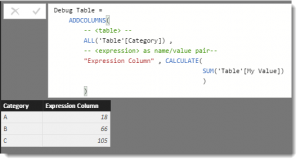### Why is CALCULATE important?

This probably traps most people when using the RANKX function in a calculated measure and I will try to explain why this is important.  The CONCATENATEX function is useful for demonstrating why the CALCULATE function is important.  Lets consider two identical measures.  One with a CALCULATE and the other without.  Otherwise, these two calculated measures are the same.

I have used the term “row context” in the name of each measure, because this is the effect the CALCULATE has on the data visible to the AggX function.

Here is the result of the two new measures when used on a visual along with the ‘Table'[Category] field.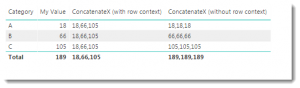### RANKX Without CALCULATE

The third column uses the CALCULATE function, while the forth column does not.

Why does the top row of the fourth column show “18,18,18” ?  There are three values because the AggX function loops the same number of times as there are rows in the <table> used as the first parameter of the AggX function.  That part is easy.  The 18 is the output of the SUM(‘Table'[My Value]) being filtered by the Category = “A” filter context from the visual.  The next loop inside the AggX function has the same filter context rules in place, so therefore produces the same result of 18.  Finally the third loop inside the AggX function also produces the same result because nothing has changed.

If we apply the pseudo logic used, this list of three 18’s is now sorted, and the value generated for the <expression> at step two (which is also 18) is then used to find the position in the list.

So with no CALCULATE, you are likely to always see a bunch of 1’s as a result.

### RANKX With CALCULATE

The third column uses CALCULATE and the list of values in the top row looks much better (18, 66 & 105).  This is because the CALCULATE function converts the value in the column of the <table> generated by the ALL function to a filter for the <expression>.  Or…… in the first loop of the AggX function the <expression> is now running the SUM(‘Table'[My Value]) over rows in ‘Table’ but are now filtered to where the Category matches the first Category from our three row table (which is “A”).  The second loop of the AggX iterator is now filtering by the “B” from the second row of the <table> and the SUM function returns 66.  The final loop of the AggX function is now using the “C” from the third row of the table.

By converting the row context to filter context the CALCULATE function eventually exposes a different set of rows to the SUM function used in the <expression>.

Note: if you use another calculated measure as your <expression>, this behaves as if it was automatically wrapped with a CALCULATE function.

So :

is the same as

so long as the following calculated measure exists in your model

### Ranking by Sub Category

Hopefully this now means the process of writing a calculated measure to show a ranking for a sub-category within a category becomes a little easier.

The final code is :

For debugging, I will also add a calculated measure that uses the CONCATENATEX AggX function.  The [ConcatenateX by Sub Category] calculated measure (below) is the same as the [Ranking by Sub Category] calculated measure (above) apart from the delimiter added to the  [ConcatenateX by Sub Category]  measure.

When used in a visual, we see the following: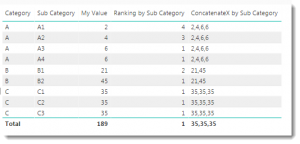The ALL Function has been extended to now include two columns.  The effect of this is to generate the following 9 row table: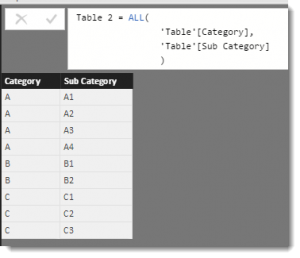The ALL Function is wrapped inside a FILTER function, which will restrict this 9 row table down to a smaller set depending on the output of the MAX(‘Table'[Category]) expression.   The effect of the FILTER is more obvious in the [ConcatenateX by Sub Category] output.  The top rows show the AggX function shows the X function is performing four internal loops and we can see the values being considered (2,4,6,6).

Whereas the measure shows (21,45) for lines where the Category is B.  This tells us the AggX function performed two iterations and we can see the values generated by our <expression> for each of the two loops.

This is how the FILTER function can be used to separate the sub category rows for Category A from those for Category B.

### Breaking Ties

As explained in Part 1. when two or more <expressions> return the same value for RANKX, it cannot break the tie.  The only way to do this is to extend the <expression> to use values from another column to help break the tie.

My data has no ties when grouped by Category, but does have some ties when grouped by Sub Category.  To split ties using the [Date] data (which I know is unique in this data-set)

The additional code is line 10, which extends the <expression> using a technique that makes the result of each <expression> unique without upsetting the initial sort order.

The same code using CONCATENATEX as the AggX function is:

With the result as shown: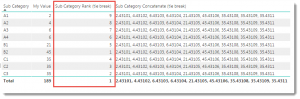### Troubleshooting

If you are still struggling with using RANKX inside a calculated measure, check the following

• Have you used the ALL function as part of the first parameter
• Use a calculated table to check the content of the <table> parameter
• Use CONCATENATEX in place of RANKX to help understand the iterations of the AggX function.  This will help show the number of loops AND the values generated by each loop
• Check to see if you are using CALCULATE appropriately.

The final step in this series will look at the optional third parameter of RANKX.

Phil is Microsoft Data Platform MVP and an experienced database and business intelligence (BI) professional with a deep knowledge of the Microsoft B.I. stack along with extensive knowledge of data warehouse (DW) methodologies and enterprise data modelling. He has 25+ years experience in this field and an active member of Power BI community.

## 7 thoughts on “How to use RANKX in DAX (Part 2 of 3 – Calculated Measures)”

•Jeff Weir says:

Great stuff, Phil.

•Ben Mosbeh Mohamed Slim says:

Explicit and step by step pedagogical methodology. I loved the debugging way, comcatenateX.
What I don’t get it is the MAX(‘Table'[Category]) , what means max of a string column ???
Thanks phil bfor sharing

•Lutz says:

I have a scenario where a particular Category needs to be excluded from the ranking. So I tried
RANKX (
FILTER(
ALL(
‘Table'[Category],
‘Table'[Sub Category]
),
and(‘Table'[Category] = MAX(‘Table'[Category],’Table'[Category] “C”)
)
),
CALCULATE(SUM(‘Table'[My Value]))
)

But that does not produce the expected result. How to I indicate the correct context so that this additional filter gets taken into account?

•Lucius says:

Thanks this is super useful and very detailed walkthrough.
However the rank by sub category expression works in powerBi but does not work in excel powerpivot, i guess it has to do with the MAX expression returning an error.

•Vivek Devalkar says:

I have a requirement where I need to show category and sub-category and amount in a table but the sort should be based on ranking by Amount at Category level and then sub-category level.
e.g. In your sample data above, all Category C values will show up first, then Category B then Category A since Total Value for Category C ? Category B > Category A
Can that be achieved in DAX?